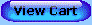For a lifetime of learning fun!

If you are unable to see the blue navigation buttons below, go to Contents for text links.All Chapters include: Chapter Review and Algebra Review

Introduction
Inductive Reasoning

Chapter 1: An Introduction to Geometry

1.1 Lines in Designing a City
1.2 Angles in Measuring the Earth
1.3 Polygons and Polyhedra: Pyramid Architecture
1.4 Constructions: Telling Time with Shadows
1.5 We Can't Go On Like This

Chapter 2: The Nature of Deductive Reasoning
2.1 Conditional Statements
2.2 Definitions
2.3 Direct Proof
2.4 Indirect Proof
2.5 A Deductive System
2.6 Some Famous Theorems of Geometry

Chapter 3: Lines and Angles
3.1 Number Operations and Equality
3.2 The Ruler and Distance
3.3 The Protractor and Angle Measure
3.4 Bisection
3.5 Complementary and Supplementary Angles
3.6 Linear Pairs and Vertical Angles
3.7 Perpendicular and Parallel Lines

Chapter 4: Congruent Triangles
4.1 Coordinates and Distance
4.2 Polygons and Congruence
4.3 ASA and SAS Congruence
4.4 Congruence Proofs
4.5 Isosceles and Equilateral Triangles
4.6 SSS Congruence
4.7 Constructions

Chapter 5: Inequalities
5.1 Properties of Inequality
5.2 The Exterior Angle Theorem
5.3 Triangle Side and Angle Inequalities
5.4 The Triangle Inequality Theorem

Chapter 6: Parallel Lines
6.1 Line Symmetry
6.2 Proving Lines Parallel
6.3 The Parallel Postulate
6.4 Parallel Lines and Angles
6.5 The Angles of a Triangle
6.6 Perimeters and Areas of Similar Figures

7.2 Parallelogram and Point Symmetry
7.3 More on Parallelograms
7.4 Rectangles, Rhombuses, and Squares
7.5 Trapezoids
7.6 The Midsegment Theorem

Chapter 8: Transformations
8.1 Transformations and Symmetry
8.2 Reflections
8.3 Isometries and Congruence
8.4 Transformations and Symmetries

Midterm Review

Chapter 9: Area
9.1 Area
9.2 Squares and Rectangles
9.3 Triangles
9.4 Parallelograms and Trapezoids
9.5 The Pythagorean Theorem

Chapter 10: Similarity
10.1 Ratio and Proportion
10.2 Similar Figures
10.3 The Side-Splitter Theorem
10.4 The AA Similarity Theorem
10.5 Proportions and Dilations
10.6 Perimeters and Areas of Similar Polygons

Chapter 11: The Right Triangle
11.1 Proportions in a Right Triangle
11.2 The Pythagorean Theorem Revisited
11.3 Isosceles and 30°-60° Right Triangles
11.4 The Tangent Ratio
11.5 The Sine and Cosine Ratios
11.6 Slope
11.7 The Laws of Sines and Cosines

Chapter 12: Circles
12.2 Tangents
12.3 Central Angles and Arcs
12.4 Inscribed Angles
12.5 Secant Angles
12.6 Tangent Segments and Intersecting Chords

Chapter 13: The Concurrence Theorems
13.1 Triangles and Circles
13.3 Incircles
13.4 The Centroid of a Triangle
13.5 Ceva's Theorem
13.6 Napoleon's Discovery and Other Surprises

Chapter 14: Regular Polygons and the Circle
14.1 Regular Polygons
14.2 The Perimeter of a Regular Polygon
14.3 The Area of a Regular Polygon
14.4 From Polygons to Pi
14.5 The Area of a Circle
14.6 Sectors and Arcs

Chapter 15: Geometric Solids
15.1 Lines and Planes in Space: Solid Geometry as a Deductive System
15.2 Rectangular Solids
15.3 Prisms
15.4 The Volume of a Prism
15.5 Pyramids
15.6 Cylinders and Cones
15.7 Spheres
15.8 Similar Solids
15.9 The Regular Polyhedra

Chapter 16: Non-Euclidean Geometries
16.1 Geometry on a Sphere